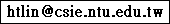## Machine Learning, Fall 2013, Project

### Data

Each line of the data set is a vector in the LIBSVM sparse format
```yn i:(xn)_i
```
where (xn)_i means the i-th feature value of xn, and features with value 0 does not need to be listed. The test set has all the yn filled with 0. The features are the intensity (gray scale) of each pixel in the images. The images are all of width 105 and height 122. For all 1<=i<=12810, the i-th feature is the intensity of the pixel at (row, column)=( (i-1)/105, ((i-1) mod 105)). That is, the feature vector is a flattened image array. The intensity is of range [0,1], where a higher value indicates a darker pixel. The labels indicate 12 classes: 1=鼠, 2=牛, 3=虎, 4=兔, 5=龍, 6=蛇, 7=馬, 8=羊, 9=猴, 10=雞, 11=狗, 12=豬.

 Last updated at CST 01:21, February 17, 2015 Please feel free to contact me: